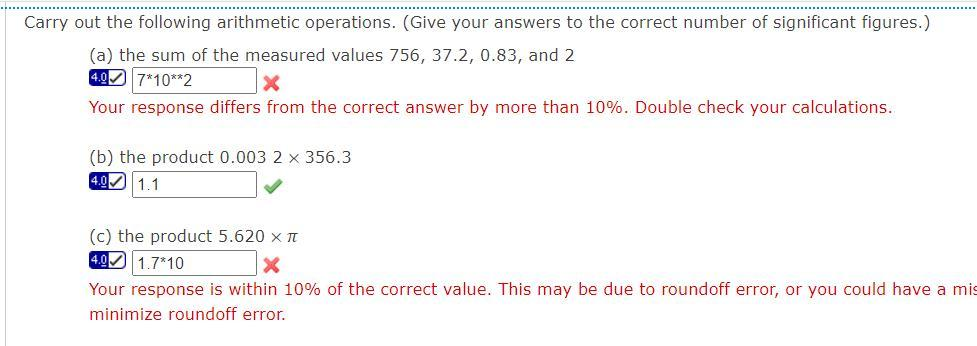# Question Solved1 AnswerCarry out the following arithmetic operations. (Give your answers to the correct number of significant figures.) (a) the sum of the measured values 756, 37.2, 0.83, and 2 4.07*10**2 X Your response differs from the correct answer by more than 10%. Double check your calculations. (b) the product 0.003 2 x 356.3 4.0 1.1 (c) the product 5.620 XT 4.9 1.7*10 Your response is within 10% of the correct value. This may be due to roundoff error, or you could have a mis minimize roundoff error.Transcribed Image Text: Carry out the following arithmetic operations. (Give your answers to the correct number of significant figures.) (a) the sum of the measured values 756, 37.2, 0.83, and 2 4.07*10**2 X Your response differs from the correct answer by more than 10%. Double check your calculations. (b) the product 0.003 2 x 356.3 4.0 1.1 (c) the product 5.620 XT 4.9 1.7*10 Your response is within 10% of the correct value. This may be due to roundoff error, or you could have a mis minimize roundoff error.
More
Transcribed Image Text: Carry out the following arithmetic operations. (Give your answers to the correct number of significant figures.) (a) the sum of the measured values 756, 37.2, 0.83, and 2 4.07*10**2 X Your response differs from the correct answer by more than 10%. Double check your calculations. (b) the product 0.003 2 x 356.3 4.0 1.1 (c) the product 5.620 XT 4.9 1.7*10 Your response is within 10% of the correct value. This may be due to roundoff error, or you could have a mis minimize roundoff error.